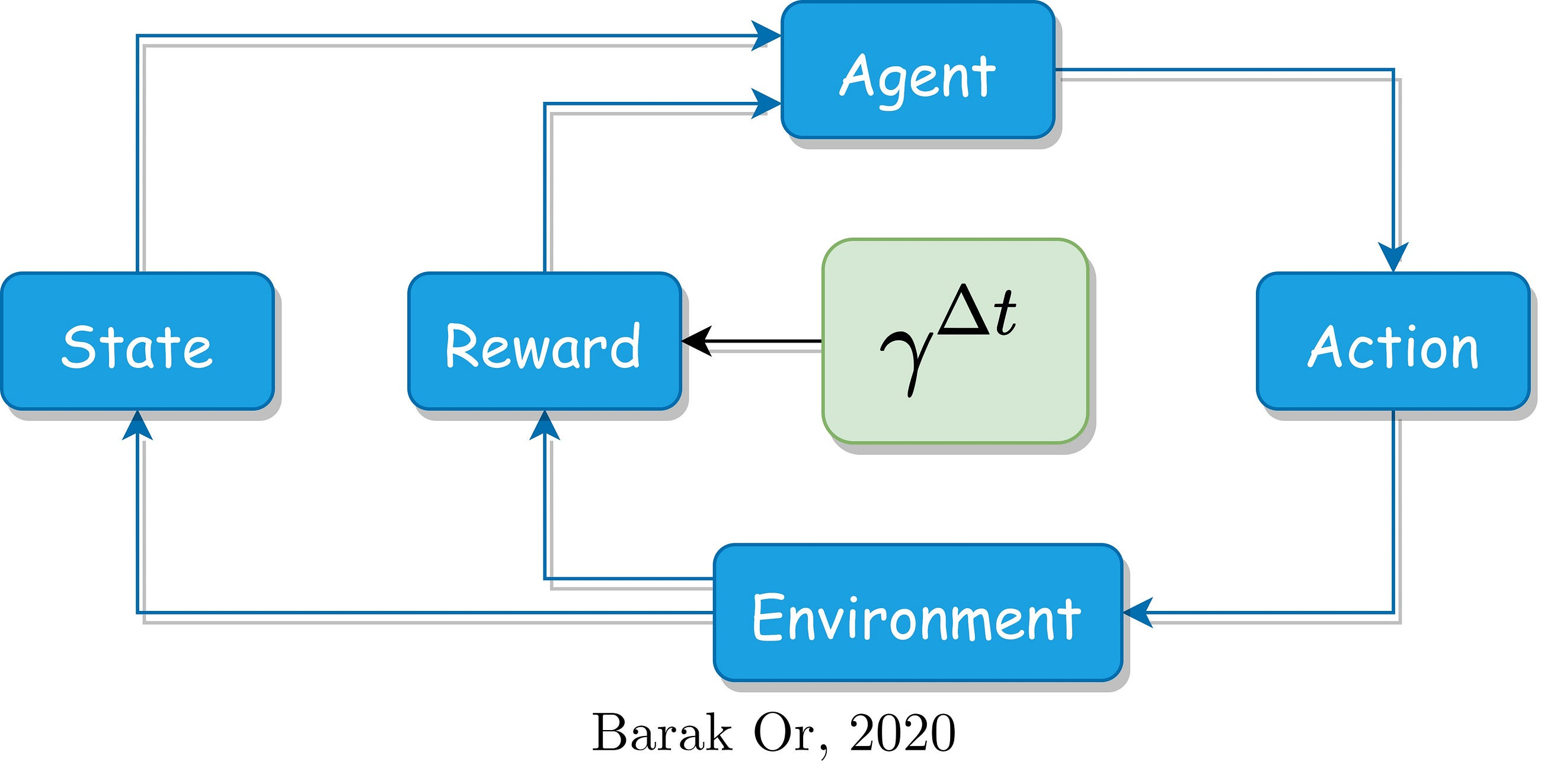# Penalizing the Discount Factor in Reinforcement Learning

Original article was published by Barak Or on Artificial Intelligence on Medium# Penalizing the Discount Factor in Reinforcement Learning

The reinforcement learning field is used in many robotics problems and has a unique mechanism. This post deals with the key parameter I found as a high influence: the discount factor. It discusses the time-based penalization to achieve better performances.

I assume that if you land on this post, you are already familiar with the RL terminology. If it is not the case, then I highly recommend these blogs which provide a great background, before you continue: Intro1 and Intro2.

# What is the role of the discount factor in RL?

The discount factor, 𝛾, is a real value ∈ [0, 1], cares for the rewards agent achieved in the past, present, and future. In different words, it relates the rewards to the time domain. Let’s explore the two following cases:

1. If 𝛾 = 0, the agent care for his first reward only.
2. If (𝛾 = 1), the agent care for all future rewards.

Generally, the designer should predefine the discount factor for the scenario episode. This might raise many stability problems and can be ended without achieving the desired goal. However, by exploring some parameters many problems can be solved with converged solutions. For further reading on the discount factor and the rule of thumb for selecting it for robotics applications, I recommend reading: resource3.

# Why penalize it?

Once the designer chooses the discount factor, it is uniform for the entire scenario, which is not the optimal case for continuous-discrete problems (and more, but let’s focus on this). Robotic dynamic is a continuous process, that we observe through various noisy sensors and by processing its information in a discrete manner (computers after all…). So, we solve a continuous problem by using discrete tools. As such numerical errors are involved. Moreover, the various sensors are corrupted with their noise which adds built-in errors. Lastly, the dynamic model we assumed (for example the states we define) is also suffering from uncertainty and includes additional errors.

Hence, by assuming a uniform discount factor we assume a uniform behavior of these error sources, which are non-uniform-behaviors. Compensating for these issues can be done by penalizing the discount factor and weigh the achieved rewards accordingly.

# Penalizing with respect to the sampling time

One common way to penalize the discount factor with respect to the sampling time (defined as the elapsed time between two successive measurements) is explained with the following example:

As the sampling interval is small, the discount factor stays the same, and when the sampling interval is large, such that a long time passes between two successive measurements, the sampling interval is changed accordingly. Remember, the discount factor lies between 0 and 1, so a large sampling interval is translated to a small discount factor (and vice versa). The formula to update the discount factor is just a suggestion to demonstrate the idea, as many other forms can be adapted.

# An Algotrding example

Consider an Algo-trading scenario where the investor (agent) controls his hedging strategy (action) in the trading market (environment), where the stock prices (states) are changed. If a long time passes since the occurrence of the latest investment, the reward cannot be modeled the same, as many changes might happen during that time.

That’s it…hope you enjoyed reading this post! please feel free to reach me out for further questions/discussion,

Barak

www.barakor.com